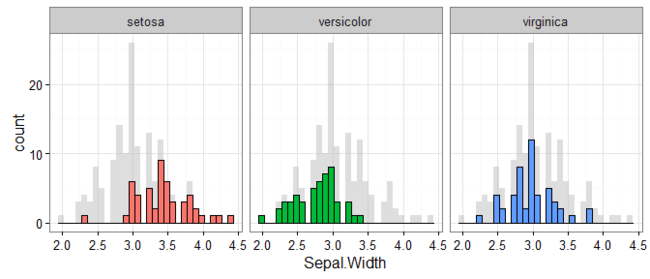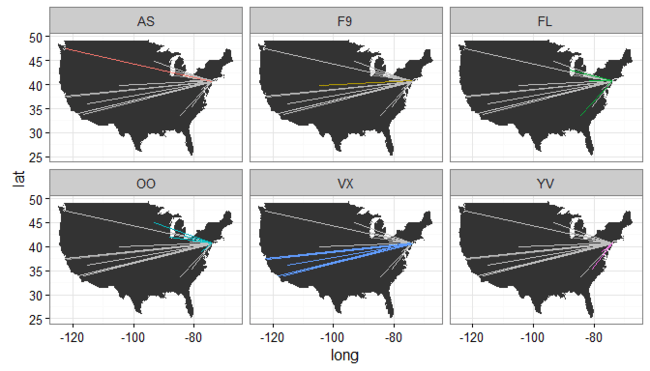[This article was first published on blogR, and kindly contributed to R-bloggers]. (You can report issue about the content on this page here)
Want to share your content on R-bloggers? click here if you have a blog, or here if you don't.

This tweet by mikefc alerted me to a mind-blowingly simple but amazing trick using the ggplot2 package: to visualise data for different groups in a facetted plot with all of the data plotted in the background. Here’s an example that we’ll learn to make in this post so you know what I’m talking about:## Credit where credit’s due

Before continuing, I’d be remiss for not mentioning that the origin of this ingenious suggestion is Hadley Wickham. The tip comes in his latest ggplot book, for which hardcopies are available online at places like Amazon, and the code and text behind it are freely available on Hadley’s Github at this repository.

## Some motivating examples

Let’s start with some examples that explain just why I’m so excited about this trick. Consider wanting to plot the results shown in the example above. That is, for the `iris` data set (that comes with R), we want to plot a histogram of the `Sepal.Width` variable, but separately for each flower `Species`. Let’s start by creating the histogram of all the data:

```library(ggplot2)
ggplot(iris, aes(x = Sepal.Width)) +
geom_histogram()
```So how can we present this separately for each of the flower `Species`? Well, here are two possibilities…

Coloured stacking via `fill`:

```ggplot(iris, aes(x = Sepal.Width, fill = Species)) +
geom_histogram()
```Or separating the results into panels with `facet_wrap()`:

```ggplot(iris, aes(x = Sepal.Width)) +
geom_histogram() +
facet_wrap(~ Species)
```There’s certainly nothing wrong with these options, but there’s always room for improvement.

## General idea for adding background data

As the first example showed, a nice improvement (and the focus of the post) is to add the complete data set in the background of each facet panel. This way, it’s easy to interpret the data for any group relative to all other groups in the data set.

The following pseudo code describes a generic approach for implementing this trick:

```ggplot(data = full_data_frame, aes(...)) +
geom_*(data = data_frame_without_grouping_var, colour/fill = "neutral_color") +
geom_*() +
facet_wrap(~ grouping_var)
```

This pseudo code will become clearer as we try some examples. For the moment, the important thing to recognise is that the first geom layer we’re plotting uses data without the grouping variable, and will appear in some neutral colour. On top of this, we add the same sort of geom, but with the group data highlighted (e.g., with different colours). Finally, we facet the plot based on our grouping variable.

Let’s try it out with some examples.

## Histogram example

We’ll start by creating something similar to the example shown at the beginning.

```d <- iris        # Full data set
d_bg <- d[, -5]  # Background Data - full without the 5th column (Species)

ggplot(d, aes(x = Sepal.Width)) +
geom_histogram(data = d_bg, fill = "grey") +
geom_histogram() +
facet_wrap(~ Species)
```The above plot is a good start. It’s already easy to see how the distribution of each `Species` fits in with the complete data set.

So how does this work? The first important point is that we have a full data set that includes the variable by which to group our data (`d` with `Species`), and a background data set without this variable (`d_bg` without `Species`).

The `ggplot()` command sets up a general canvas with our full data set.

We then plot a `geom_histogram()` using the background data (`d_bg`) and `fill` it grey so as to give it a neutral appearance. It makes use of the `aes()` command within `ggplot()`, thus plotting the data we want.

On top of this, we plot another `geom_histogram()`. Because we haven’t specified a data set, it will use `d`, which is specified in `ggplot()`. This time, it will plot in the default colour, black.

Finally, we `facet_wrap()` the plot by `Species`. Because `Species` is not in the background data set, the output of our first `geom_histogram()` is replicated across all the panels. But because it is in the full data set used to plot the second `geom_histogram()` layer, this data gets split up.

Below is the code that makes this all a bit prettier by adding the colours and visual touch-ups that appear in the first example.

```d <- iris        # Full data set
d_bg <- d[, -5]  # Background Data - full without the 5th column (Species)

ggplot(d, aes(x = Sepal.Width, fill = Species)) +
geom_histogram(data = d_bg, fill = "grey", alpha = .5) +
geom_histogram(colour = "black") +
facet_wrap(~ Species) +
guides(fill = FALSE) +  # to remove the legend
theme_bw()              # for clean look overall
```The code used to produce this is similar to the earlier pseudo code but with a few tweaks to improve the overall look of the plot. For visual improvements:

• The background data is made transparent with `alpha = .5`.
• The second `geom_histogram()` layer makes use of the `fill = Species` in `ggplot()` to color each group differently. This adds a legend which is later removed with `guides(fill = FALSE)`.
• The coloured bars are then outlined with `colour = "black"`
• Clean overall look with `theme_bw()`

## Points example

Let’s take a look at another example with the same data set.

Here it is following the pseudo code without any special tweaking:

```ggplot(d, aes(x = Sepal.Width, y = Sepal.Length)) +
geom_point(data = d_bg, colour = "grey") +
geom_point() +
facet_wrap(~ Species)
```And here it is with some visual improvements:

```ggplot(d, aes(x = Sepal.Width, y = Sepal.Length, colour = Species)) +
geom_point(data = d_bg, colour = "grey", alpha = .2) +
geom_point() +
facet_wrap(~ Species) +
guides(colour = FALSE) +
theme_bw()
```This example plots the points of `Sepal.Width` against `Sepal.Length` separately for each `Species`. The logic is the same as above:

• Background data without the grouping variable (`Species`) plotted first in a neutral colour.
• Full data set plotted on top of this.
• The plot is facetted by the grouping variable, which only appears in the full data set.

## Map example

Here’s one final example I’d like to share with you:

```library(nycflights13)
library(dplyr)

usa_map <- map_data("usa")

airports <- read.csv("https://raw.githubusercontent.com/jpatokal/openflights/master/data/airports.dat", stringsAsFactors = FALSE, header = FALSE)
airports <- airports[, c(5, 7, 8)]
names(airports) <- c("code", "lat", "long")
orig <- airports %>% dplyr::rename(origin = code, long_o = long, lat_o = lat)
dest <- airports %>% dplyr::rename(dest = code, long_d = long, lat_d = lat)

d <- flights %>%
left_join(orig) %>%
left_join(dest) %>%
filter(carrier %in% c("AS", "F9", "OO", "YV", "VX", "FL"))

d_bg <- d %>% select(-carrier)

ggplot(d) +
geom_polygon(data = usa_map, aes(long, lat, group = region)) +
geom_segment(data = d_bg, colour = "grey", alpha = .7,
aes(x = long_o, y = lat_o,
xend = long_d, yend = lat_d)) +
geom_segment(aes(x = long_o, y = lat_o,
xend = long_d, yend = lat_d,
colour = carrier)) +
facet_wrap(~ carrier) +
guides(colour = FALSE) +
theme_bw()
```This plot presents the flight paths for six airline carriers that fly in/out of New York (data from the nycflights13 package). All flight paths appear in the background of each plot, and the flight paths of the relevant carrier are highlighted.

The code that comes before `ggplot()` does all the data prep (which you can do with the appropriate packages installed). If you run the code yourself, you’ll find that you end up with a full data set, `d`, and a background data set without the flight carriers, `d_bg`. The plot itself is a bit different because we need to place the map in the background, but it’s the same logic as presented earlier.

## Sign off

Thanks for reading and I hope this was useful for you.

For updates of recent blog posts, follow @drsimonj on Twitter, or email me at [email protected] to get in touch.

If you’d like the code that produced this blog, check out the blogR GitHub repository.